Math Olympiad Test: Surface Area and Volume- 3

# Math Olympiad Test: Surface Area and Volume- 3 - Class 10

Test Description

## 10 Questions MCQ Test Olympiad Preparation for Class 10 - Math Olympiad Test: Surface Area and Volume- 3

Math Olympiad Test: Surface Area and Volume- 3 for Class 10 2023 is part of Olympiad Preparation for Class 10 preparation. The Math Olympiad Test: Surface Area and Volume- 3 questions and answers have been prepared according to the Class 10 exam syllabus.The Math Olympiad Test: Surface Area and Volume- 3 MCQs are made for Class 10 2023 Exam. Find important definitions, questions, notes, meanings, examples, exercises, MCQs and online tests for Math Olympiad Test: Surface Area and Volume- 3 below.
 1 Crore+ students have signed up on EduRev. Have you?
Math Olympiad Test: Surface Area and Volume- 3 - Question 1

### An inverted cone of vertical height 12 cm and the radius of base 9 cm contains water to a depth of 4 cm. Find the area of the interior surface of the cone not in contact with the water. [Use π = 22/7]

Detailed Solution for Math Olympiad Test: Surface Area and Volume- 3 - Question 1

Since, Δ OAB ~ Δ OCD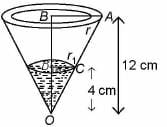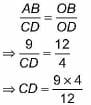⇒ CD = 3 cm
Slant height of cone (/) =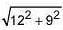= 15 cm
Curved surface area of the conical pot will be S = π × r × l = 135π cm2
Let l' be the slant height occupied by water of depth h' = 4cm
So l'/l = h'/h
⇒ l' = 4/12 × 15 = 5cm
r' = √(l'- h'2)=√(52 - 42) = 3cm
Curved surface area of water cone
= π × r' × l' = 15π
So area of interior surface of the cone not in contact with water will be
= (135 - 15)π cm2 = 377.14 cm2

Math Olympiad Test: Surface Area and Volume- 3 - Question 2

### If the radii of the circular ends of bucket in the form of frustum are 28 cm and 7 cm and the height is 45 cm. The capacity of the bucket is _____.

Detailed Solution for Math Olympiad Test: Surface Area and Volume- 3 - Question 2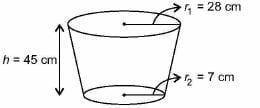Volume of frustum/bucket =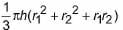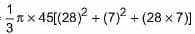= 48510 cm3

Math Olympiad Test: Surface Area and Volume- 3 - Question 3

### A cylinder, whose height is two-thirds of its diameter, has the same volume as a sphere of radius 4 cm. Calculate the radius of the base of the cylinder.

Detailed Solution for Math Olympiad Test: Surface Area and Volume- 3 - Question 3

Let the radius of the cylinder be 2/3 diameter
So, diameter of the cylinder = 2r
∴ Height of the cylinder = 2/3(2r) = 4r/3
Volume of the cylinder = Volume of the sphere of radius 4 cm
⇒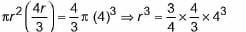⇒ r3 = 43 ⇒ r = 4 cm.

Math Olympiad Test: Surface Area and Volume- 3 - Question 4

The number of solid spheres, each of diameter 6 cm that could be moulded to form a solid metal cylinder of height 45 cm and diameter 4 cm, is ____.

Detailed Solution for Math Olympiad Test: Surface Area and Volume- 3 - Question 4

Volume o f one solid sphere =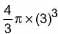Volume of cylinder = πr2h = π x 4 x 45

Now, Number of spheres x Volume of one sphere = Volume of cylinder

⇒ Number of spheres x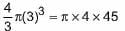⇒ Number of spheres = 45/9 = 5

Math Olympiad Test: Surface Area and Volume- 3 - Question 5

The height of a cone is 30 cm. A small cone is cut off at the top by a plane parallel to the base. If its volume be 1/27 of the volume of the given cone, at what height above the base is the section made?

Detailed Solution for Math Olympiad Test: Surface Area and Volume- 3 - Question 5

Let OC = h and AB = r

Now, Δ OAB ~ Δ OCD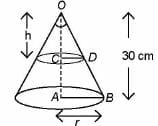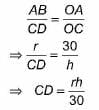Volume of smaller cone = 1/27 (Volume of whole cone)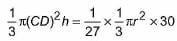⇒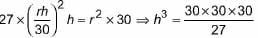h3 = 10 x 10 x 10

⇒ h = 10 cm.

Required height, AC = 30 - 10 = 20 cm

Math Olympiad Test: Surface Area and Volume- 3 - Question 6

Study the question and the statements given below and decide which of the statement(s) is/are necessary to answer the question.
What is the capacity of the cylindrical tank?
I. The area of the base is 61,600 sq. cm.
II. The height of the tank is 1.5 times the radius.
III. The circumference of base is 880 cm.

Detailed Solution for Math Olympiad Test: Surface Area and Volume- 3 - Question 6

Volume of cylindrical tank = πr2h

So, we need radius and height.
Radius can be found from either statement I or III and height from statement II.

Math Olympiad Test: Surface Area and Volume- 3 - Question 7

Find the maximum volume of a cone that can be carved out of a solid hemisphere of radius r.

Detailed Solution for Math Olympiad Test: Surface Area and Volume- 3 - Question 7

To cut out maximum area, radius of the cone = radius of the hemisphere = r and height of the cone = radius of the hemisphere = r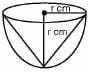∴ Volume of the cone = 1/3πr2h

= 1/3πr3

Math Olympiad Test: Surface Area and Volume- 3 - Question 8

A tent is in the shape of a right circular cylinder up to a height of 3 m and then becomes a right circular cone with a maximum height of 13.5 m above the ground. Calculate the cost of painting the inner side of the tent at the rate of ₹ 2 per m2, if the radius of the base is 14 m.

Detailed Solution for Math Olympiad Test: Surface Area and Volume- 3 - Question 8

Height of cylinder = 3 m

Height of cone = 10.5 m

Slant height of cone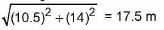Total curved surface area of tent = Curved surface area of cylinder + Curved surface area of cone

= π(2 x 14 x 3 + 14 x 17.5)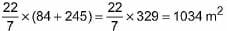Cost of painting the inner surface at the rate of ₹ 2 per m2 = 2 x 1034 = ₹ 2068

Math Olympiad Test: Surface Area and Volume- 3 - Question 9

A bucket is in the form of a frustum of a cone, its depth is 15 cm and the diameters of the top and the bottom are 56 cm and 42 cm respectively. How many litres can the bucket hold?

Detailed Solution for Math Olympiad Test: Surface Area and Volume- 3 - Question 9

Volume of bucket =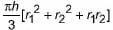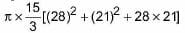= 28490 cm3

∴ Volume of bucket = 28490 cm3 = 28.49 L

Math Olympiad Test: Surface Area and Volume- 3 - Question 10

In a marriage ceremony of her daughter Poonam, Ashok has to make arrangements for the accommodation of 150 persons. For this purpose, he plans to build a conical tent in such a way that each person have 4 sq. metres of the space on ground and 20 cubic metres of air to breath. What should be the height of the conical tent?

Detailed Solution for Math Olympiad Test: Surface Area and Volume- 3 - Question 10

Total number of people = 150
Space required for each person on the ground = 4 m2
Area of base of the cone = 150 x 4
⇒ πr2 = 600 ⇒ r2 =  2100 /11
Volume of air required by 150 persons = 150 x 20.
⇒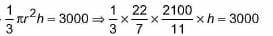⇒ 200h = 3000 ⇒ h = 15 m

## Olympiad Preparation for Class 10

11 videos|36 docs|201 tests